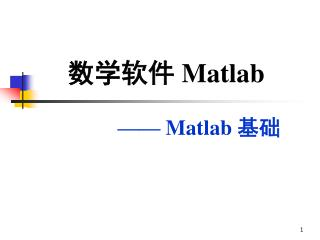DownloadDownload Presentation# 数学软件 Matlab

Download Presentation## 数学软件 Matlab

- - - - - - - - - - - - - - - - - - - - - - - - - - - E N D - - - - - - - - - - - - - - - - - - - - - - - - - - -
##### Presentation Transcript

1. 数学软件 Matlab —— Matlab 基础

2. 本讲主要内容 • Matlab 介绍 • Matlab 的基本用法

3. Matlab 介绍 Matlab Matrix Laboratory 矩阵实验室 • Matlab是一种广泛应用于工程计算及数值分析领域的新型高级语言，自 1984 年推向市场以来，经过多年的发展与竞争，现已成为国际公认的最优秀的工程应用开发环境。 • 在欧美各高等院校，Matlab 是线性代数、数值分析、数理统计、自动控制、数字信号处理、动态系统仿真、图像处理等课程的基本教学工具，已成为大学生必须掌握的基本技能之一。

4. Matlab 的发展 • Matlab 的发展 • Matlab是Matrix Labotary的缩写，最初是美国新墨西哥大学Cleve Moler教授编写的LINPACK 和EISPACK（Moler早期编写的Fortran子程序库）接口程序。 Cleve Moler John Litlle • 1983年春天， CleveMoler到Stanford大学讲学，工程师John Litlle 深为吸引，同年他和Moler, Steve Banger 一起，用 C 语言重新改写 Matlab。 • 1984年，Moler和John Litlle 等成立了 The MathWorks的公司，正式将Matlab推向市场。

5. Matlab 的发展 • 到九十年代，在国际上 30 几个数学类科技应用软件中，Matlab 在数值计算方面独占鳌头。 • 目前，Matlab 已成为世界顶尖的数学应用软件就影响而言， 至今仍然没有一个别的计算软件可与 Matlab 匹敌。

6. Matlab 的发展 • Matlab 的发行版本 1984年，Matlab 1.0 （DOS版，182K，20多个函数） 1992年，Matlab 4.0 （93年推出Windows版，加入 simulink）1994年，Matlab 4.2（得到广泛重视和应用） 1999年，Matlab 5.3（真正实现32位运算）2002年，Matlab 6.5（采用JIT加速器） 2004年，Matlab 7.0 自2006年起，Matlab每年更新两次 目前最新版为 R2012b （2012年9月发布）

7. Matlab 的功能与特点 • Matlab 是一个交互式软件系统 • 输入一条命令，立即就可以得到该命令的运行结果 • Matlab 具有很强的数值计算功能 • Matlab 以矩阵作为数据操作的基本单位， 但无需预先指定矩阵维数（动态定维） • 按照 IEEE 的数值计算标准进行计算 • 提供十分丰富的数值计算函数，方便计算，提高效率 • Matlab 命令与数学中的符号、公式非常接近， 可读性强，容易掌握

8. Matlab 的特点与功能 • Matlab 的符号计算功能 Matlab 可以进行一些常见的符号计算 • Matlab 的绘图功能 Matlab提供丰富的绘图命令，很方便实现数据的可视化 • Matlab 的编程功能 Matlab具有程序结构控制、函数调用、数据结构、输入输出、面向对象等程序语言特征，且简单易学、编程效率高

9. Matlab 的特点与功能 • Matlab 丰富的工具箱（toolbox） 根据专门领域中的特殊需要而设计的各种可选工具箱 Symbolic Math PDE Optimization Signal process Image Process Statistics Control System System Identification … … • Matlab 的 Simulink 动态仿真集成环境 提供建立系统模型、选择仿真参数和数值算法、启动仿真程序对该系统进行仿真、设置不同的输出方式来观察仿真结果等功能

10. Matlab 的基本用法 • Matlab 的启动 • 使用 Windows “开始” 菜单 • 运行 Matlab 系统启动程序 matlab • 双击 Matlab 快捷图标 • Matlab 的退出 • 在 Matlab 主窗口 File菜单中选择 Exit Matlab • 在 Matlab 命令窗口输入 exit 或 quit • 单击 Matlab 主窗口的“关闭”按钮

11. Matlab 的工作界面 当前目录 命令窗口 当前工作空间 当前目录中的文件 命令行提示符 命令 历史记录

12. Matlab 变量 • Matlab 语句的通常形式 变量 =表达式 • 表达式是用运算符将有关运算量连接起来的式子 • 计算表达式的结果，并赋给赋值号“=”左边的变量 Matlab 命令的执行：回车 • Matlab 变量的命名规则 • 以字母开头 • 后面可以跟 字母、数字 和 下划线 • 长度一般不要超过 63 个字符 • 字母区分 大小 写

13. 变量的查看 • 查看已定义的变量：who、whos • 清除变量：clear

14. 系统预定义变量 • 系统预定义的变量 注：应尽量避免给系统预定义变量重新赋值！ • 特殊变量ans

15. 复数的输入 例：z=3+4i (4 与 i 之间不能有空格) Matlab 数值运算 • Matlab 中的数与算术表达式 • 默认是双精度实数，可用浮点数或科学计数法表示 例：3, 0.4, 1.603e-12 • 浮点运算的相对误差为eps，大约为2.210-16 • 浮点数表示范围为：10-308 ～ 10308 注：复数作为矩阵元素输入时，加号两边不要加空格!

16. Matlab 数值运算 • 算术运算 • 命令分隔符 • 一行可以写多个语句，语句间用逗号或分号隔开 • 分号的作用 • 若不想在屏幕上输出结果，可以在语句最后加分号 • 续行符 • 如果语句很长，可用续行符 “…”（三个点）续行 续行符的前面最好留一个空格

17. 矩阵的输入 • Matlab 的操作对象 矩阵 • 定义矩阵：直接输入法 例： A=[1 2 3; 4 5 6; 7 8 9] • 矩阵用方括号 “[ ]” 括起 • 矩阵同一行中的元素之间用 空格 或 逗号 分隔 • 矩阵行与行之间用 分号 分开，也可以用 回车 代替

18. 矩阵元素赋值 • 矩阵元素可以是任何数值表达式 例：x=[-1.3, sqrt(3), (1+2+3)*4/5] • 矩阵元素的单独赋值 例：x(2)=6.8 例：x(5)=abs(x(1)) 动态定维功能：Matlab 会自动扩展向量的长度， 并将没有赋值的部分置零 例：x(5)=abs(x(6))??

19. 矩阵元素赋值 • 大矩阵可以把小矩阵作为其元素 例：A=[A ; 11 12 13] 在原矩阵的下方加一行 思考：如何在原矩阵的右边添加一列？ • 空矩阵 例：B=[]

20. 矩阵元素的引用 • 单个元素的引用 例：x=A(2,3)+A(1,2) • 多个元素的引用：冒号的特殊用法 • 产生一个由等差序列组成的向量 • a是首项，b是公差，c确定最后一项 • 若 b = 1，则 b和其前面的冒号可以省略 a:b:c 例：x=1:2:5 y=1:2:6 例：x=2:1:5 y=2:5 例：x=3:1:0

21. 矩阵元素的引用 • 多个元素的引用（续） 例：y=x(1:3) 例：B=A(2:3,1:3) y=A(1,1:3)

22. 矩阵元素的引用 • 多个元素的引用（续）

23. 矩阵元素的引用 • 多个元素的引用：不连续元素的选取 例：A([1,3,4], [2,3]) 例：A([1,4,3], [2,3]) • 删除指定的行或列

24. 特殊矩阵 • 特殊矩阵的生成 • Matlab 提供了一些函数，用于生成一些常见的特殊矩阵 例： A=magic(3) B=ones(4) A=magic(5) a1=diag(A) a2=diag(A,1) a3=diag(A,-2) 注意 diag的用法 a=[1,2,3,4] A1=diag(a) A2=diag(a,1) A3=diag(a,-2)

25. 常见矩阵生成函数

26. 矩阵基本运算 • 矩阵的加减：对应分量进行运算 例： A=[1,2; 3,4]; B=[5,6; 7,8] C=A+B D=B-A 参与加减运算的矩阵具有 相同的维数！ • 矩阵的普通乘法 例： A=[1,2,3; 4,5,6]; B= [2,1; 4,3] C=B*A 参与运算的矩阵须满足线性代数中矩阵相乘的原则！

27. 矩阵基本运算 • 矩阵的除法：若 A 可逆方阵，则 B/A<==>A 的逆右乘B<==>B*inv(A) 右除 A\B<==>A 的逆左乘B<==>inv(A)*B 左除 • 矩阵的幂：若 A 是方阵，p是正整数，则 A^p==>A的 p次幂，即 p个 A相乘 • 矩阵的转置与共轭转置 例： A = [1, 2-3i; 3, 1+4i] B = A' C = A.'

28. 翻转与旋转 • 矩阵的翻转与旋转 例： A = [1 2 3;4 5 6] B = fliplr(A) C = flipud(A) D = rot90(A) E = rot90(A,-1) 注意矩阵旋转与转置的区别！

29. 改变矩阵的形状 • 改变矩阵的形状：reshape(A,m,n) 将矩阵元素按 列方向 进行重新排列成一个 m×n 的新矩阵 新矩阵的元素个数必须与原矩阵元素个数相等！ 例： A=[1,2,3; 4,5,6; 7,8,9; 10,11,12] B=reshape(A,4,3) C=reshape(A,2,6) × C=reshape(A,5,2)

30. 查看矩阵的大小 • 查看矩阵的大小：size、length 例： A=[1,2,3; 4,5,6] size(A) size(A,1) size(A,2) length(A), numel(A) x=1:5; length(x)

31. Matlab帮助系统 • 如何寻找帮助 例： help size doc size • 查找命令 • 其它相关命令 更多 Matlab 命令，参见课程主页 cd、dir、more

32. 几个小技巧 • Matlab 的命令记忆功能：上下箭头键 可以先输入命令的前几个字符，再按上下键缩小搜索范围 • 命令补全功能： Tab 键 • 用 Esc 键 删除命令行 • 命令 home、clc

33. 上机要求 • 上机要求 • 将完成每题所用的命令写入一个文件，取名为 m01.m • 然后将该文件作为附件，以邮件形式发给 mathshnu@sina.com • 邮件主题为：学号-姓名 • 两个字段之间用英文状态下的减号连接 • 上机安排 座位基本固定

34. 上机作业 1、写出可以完成下列任务的Matlab命令 (函数) ： （1）Matlab中的圆周率  约等于多少？ （2）Matlab中的浮点运算相对精度是多少？ （3）如何知道命令 realmax，realmin 的具体用法？ （4）如何知道函数 max 的作用？并举例加以说明。 2、回答以下问题： （1）Matlab 是什么含义？ （2）Matlab 命令 who 与 whos 有什么区别？ （3）如何使用 Matlab 命令补全功能？ （4）Matlab 命令 clear、clc 与 home 有什么区别？

35. 上机作业 3、写出可以完成下列任务的Matlab命令 ： （1）生成 5 阶的单位阵和 8 阶均匀分布的随机矩阵 （2）生成一个列向量 x = [1, 3, 5, 7, 9, … , 99] （3）生成以 x的前 8 个元素为对角线的矩阵 A （4）生成一个与 A 同阶的正态分布的随机矩阵 B （5）计算 A 的转置 与 B 的下三角部分的乘积 C （6）删除 C 的第三行和第二列 （7）生成由 B 的第 2、4、5 行和第4、1 列组成的子矩阵 D （8）教材第 53 页，第 5 题(提示：5 (7) 可以使用 diag 来实现)